## A solar panel is used to recharge a battery. The solar panel produces 0.80 W of electrical power. The panel is 20% efficient. What is the po

Question

A solar panel is used to recharge a battery. The solar panel produces 0.80 W of electrical power. The panel is 20% efficient. What is the power input of the sunlight onto the solar panel? * 1 point 0.16 W 4.0 W 8.0 W 16.0 W

in progress 0
6 months 2021-07-16T16:30:24+00:00 1 Answers 34 views 0

Input power, Ip = 4 Watts

Explanation:

Given the following data;

Power output = 0.8 Watts

Efficiency = 20%

To find the power input of the sunlight onto the solar panel;

Mathematically, the efficiency of a machine is given by the formula;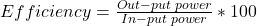Substituting into the formula, we have;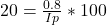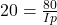Cross-multiplying, we have;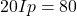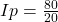Input power, Ip = 4 Watts